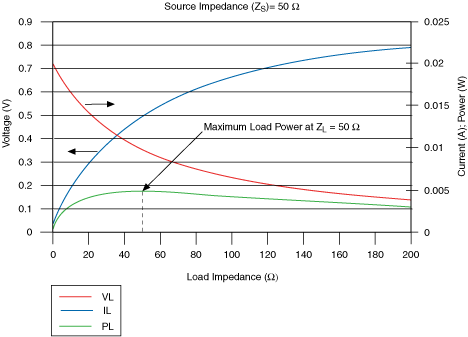# Characteristic Impedance

NI Switches Help

Edition Date: April 2015

Part Number: 375472H-01

»View Product InfoDownload Help (Windows Only)

Characteristic impedance is a transmission line parameter that determines how propagating signals are transmitted or reflected in the line. The following equation and figures represent the components of characteristic impedance.

 Z0=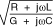where

Z0 is the characteristic impedance

L is the inductance per length

C is the capacitance per length

R is the resistance per length

G is the dielectric conductance per lengthis the frequency (radians/s)

If a transmission line is in the system, its characteristic impedance must also match the source and the load for maximum power transfer.

In an ideal, lossless transmission line, there is no series resistance or dielectric loss, as shown by the following formula:

 R = 0G = 0 => Z0 =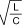(frequency independent)

The following figure shows an electrical system diagram

where

Zs is the source impedance

Z0 is the characteristic impedance of the transmission line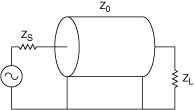The following figure is an electrical representation of a transmission line showing the components that affect characteristic impedance.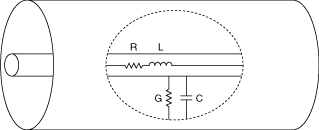Maximum power is transferred from the source to the load when both have the same impedance. This is displayed in the following graph which shows the voltage, current, and power transferred at various load impedances. The graph assumes no transmission line losses and a source impedance of 50 Ohms.